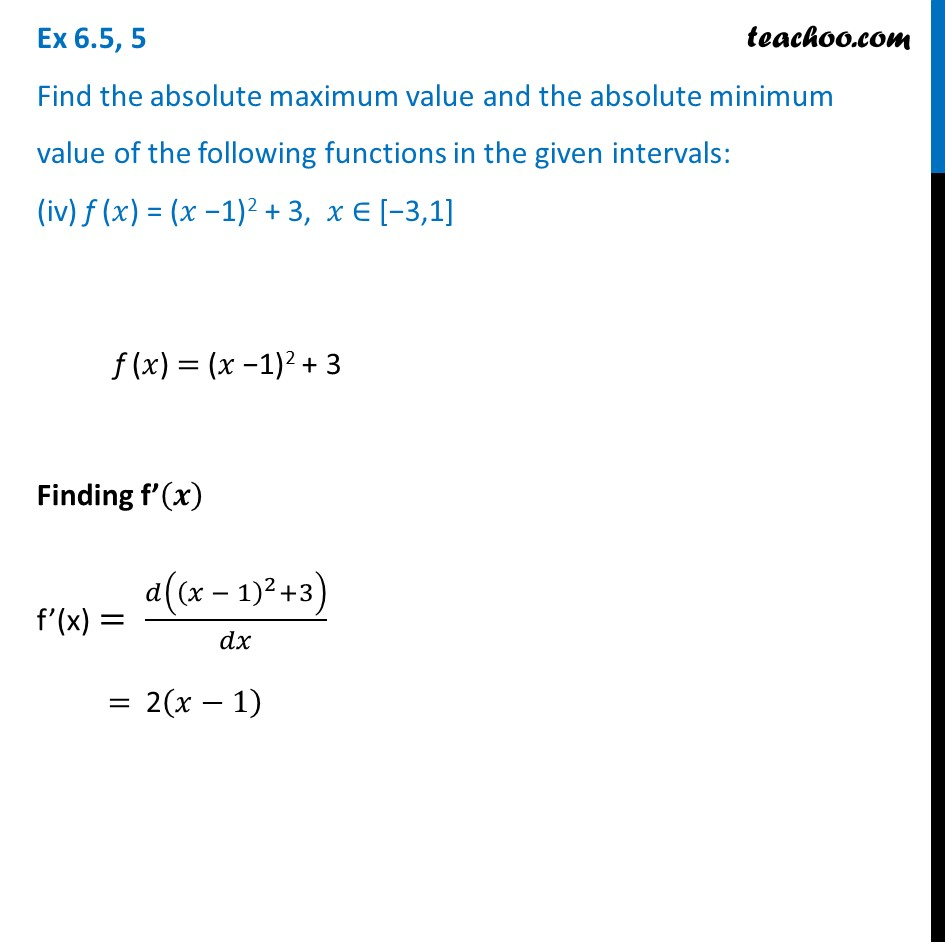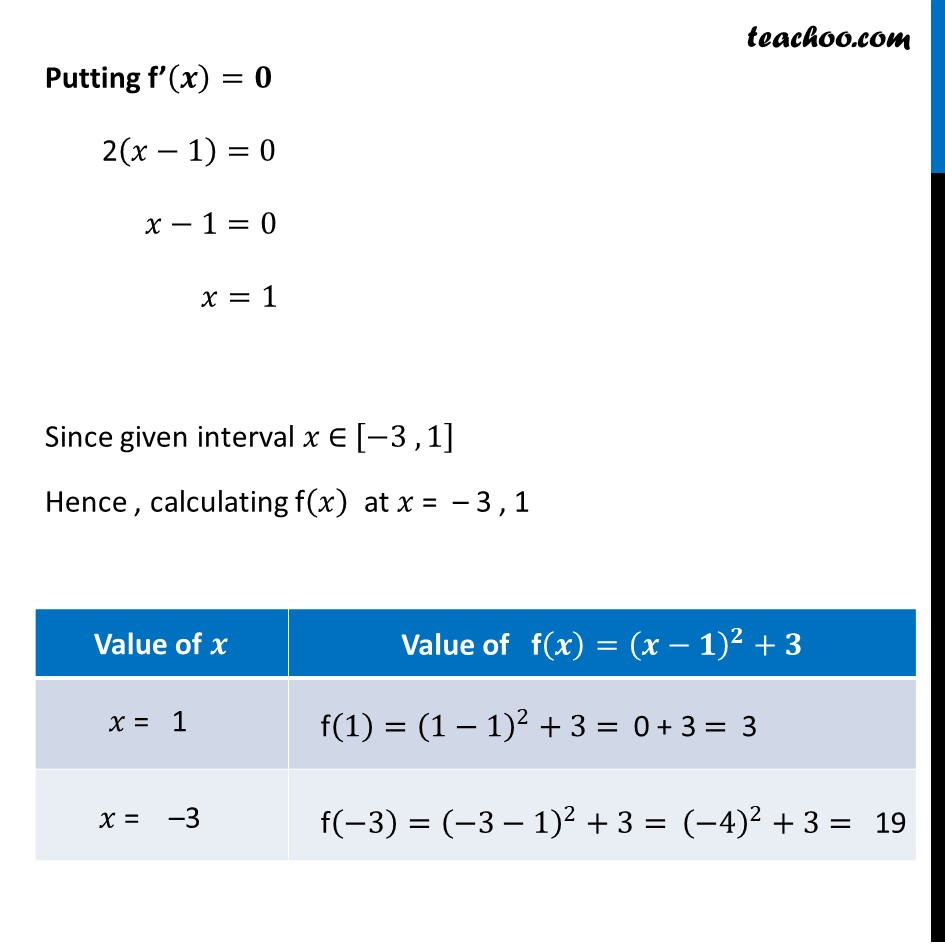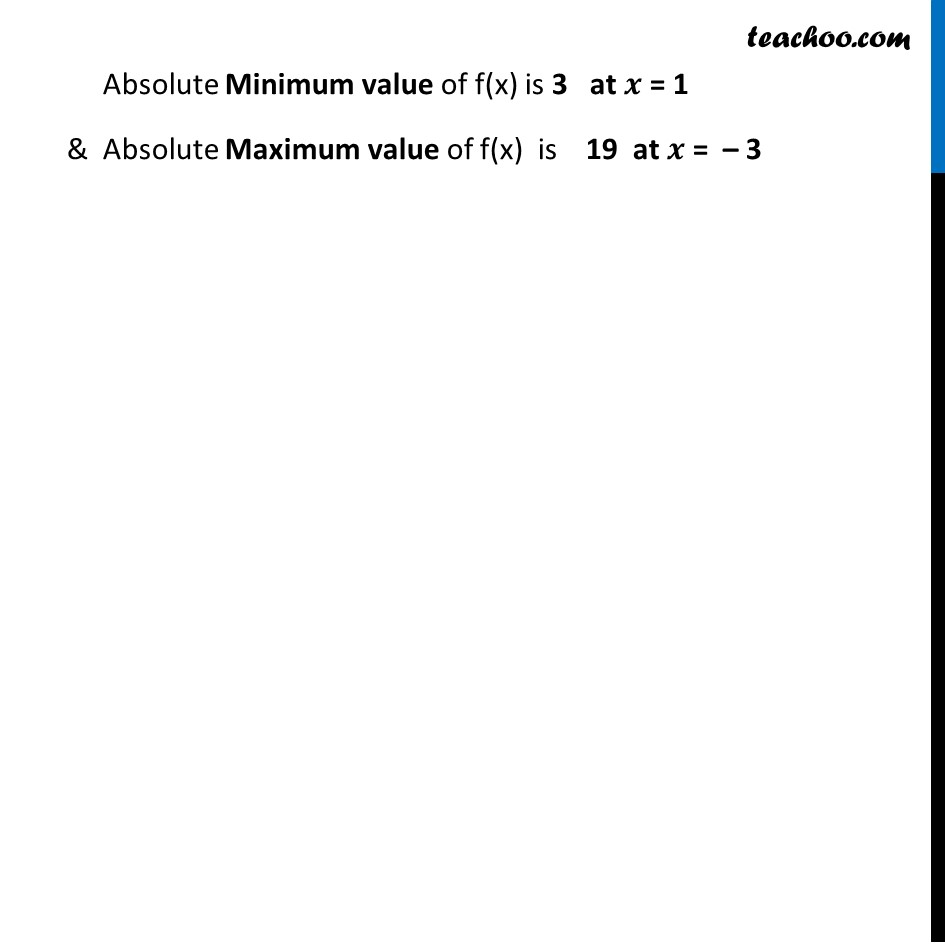Ex 6.3

Chapter 6 Class 12 Application of Derivatives
Serial order wiseLearn in your speed, with individual attention - Teachoo Maths 1-on-1 Class

### Transcript

Ex 6.3, 5 Find the absolute maximum value and the absolute minimum value of the following functions in the given intervals: (iv) f (𝑥) = (𝑥 −1)2 + 3, 𝑥 ∈ [−3,1] f (𝑥) = (𝑥 −1)2 + 3 Finding f’(𝒙) f’(x) = 𝑑((𝑥 − 1)^2+3)/𝑑𝑥 = 2(𝑥−1) Putting f’(𝒙)=𝟎 2(𝑥−1)=0 𝑥−1=0 𝑥=1 Since given interval 𝑥 ∈ [−3 , 1] Hence , calculating f(𝑥) at 𝑥 = – 3 , 1 Absolute Minimum value of f(x) is 3 at 𝒙 = 1 & Absolute Maximum value of f(x) is 19 at 𝒙 = – 3